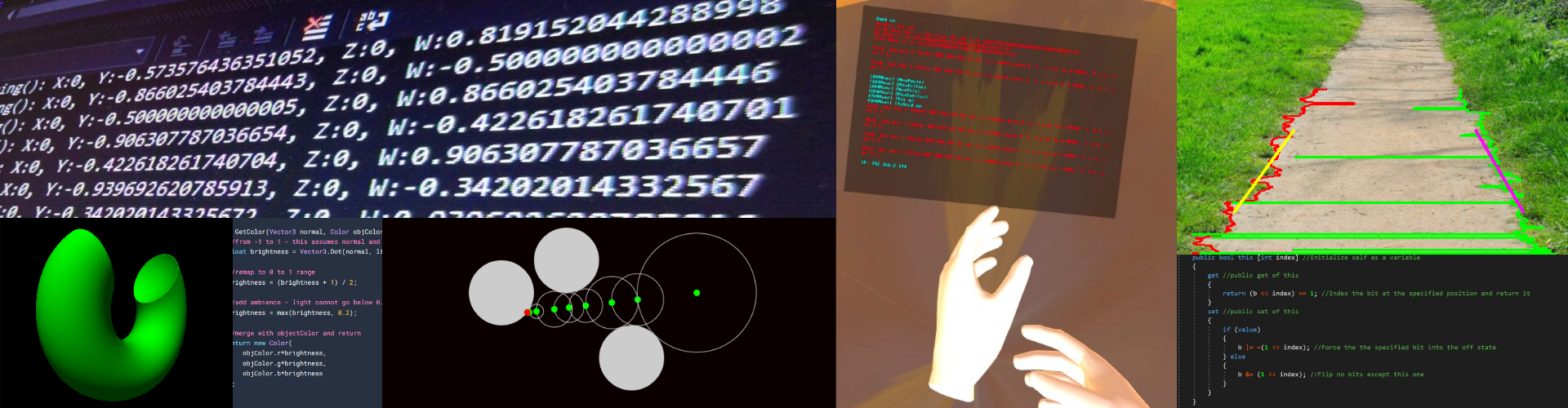# Raymarching

I decided to make another smaller project this time, but this time looking into ray marching. Below is an interactive demonstration of how ray marching would work (in 2D). To control the demonstration, use your mouse to move the ray angle and use the WASD keys to move the casting point. If it doesn’t work, use the link below or just look at the images

The idea is that using an angle, you calculate a point on the unit circle that represents the direction of the ray that you are casting. We will use this later. Then, for every object in the scene, you calculate the closest distance to the point you are casting the ray from. This distance is represented as a ring on the demonstration, with the green point as where the ray is cast from. After this, we advance the ray in the direction of the point on the unit sphere we calculated earlier multiplied by the minimum distance. It sounds a bit complicated, but maybe the example above can explain it better. There are screenshots below.

Obviously in 2D, the process is simple. However, ray marching is a 3D rendering technique. In 3D, it is a little more complicated and time consuming. Instead of just casting one ray, we need to cast one for every pixel on the screen – converting the pixel coordinates to angles and then to points on the unit sphere for the raycaster to use. After this, we should have a few spheres of block colour on the screen, if all goes well.

Now that we have this, we can add some shading. I did this using a technique I learned while writing my 3D engine. I’m not sure exactly what its called, but it gives a similar effect to phong lighting. We take the normal of the shape and the lighting and then use the dot product of them. Because its paramters are normalised (they have length 1), dot product does something special – it returns the ‘likeness’ of the vectors. For example, if they were identical, it would return 1, 90 degrees apart would return 0, opposite would return -1 (this works for every angle in between).

```Color GetColor(Vector3 normal, Color objColor) {
//from -1 to 1 - this assumes normal and lightDirection are normalized
float brightness = Vector3.Dot(normal, lightDirection);

//remap to 0 to 1 range
brightness = (brightness + 1) / 2;

//add ambience - light cannot go below 0.2 so shape is always visible
brightness = max(brightness, 0.2);

//merge with objectColor and return
return new Color(
objColor.r*brightness,
objColor.g*brightness,
objColor.b*brightness
);
}```

Now that we have this, we can apply shading to the sphere. We can also do fancy effects such as where the colour of the object is its normal.

Ok! Now that that’s done, we can add some other objects such as toruses and cubes. I’m going to be honest here – the maths behind this is so confusing (everything is a distance function for the nearest point) that I ended up writing the sphere one and just copying everything else from a website (thanks https://iquilezles.org/www/articles/distfunctions/distfunctions.htm). We can move the object by telling the distance function that the ray is coming from a different point.

Now here’s where it gets interesting. Because the shapes are described as distance functions, we can use boolean operators on them such as union, intersect and difference. Union means combination of the two – this is like drawing both shapes separately. Intersect only draws the area where both shapes meet (i.e. intersect), and difference cuts out a hole in one object in the shape of the other. You can see these below.

The way we do this is using `min` and `max` functions. When using `min`, this gets the closest object and thus performs a union operation. When using `max`, this gets the furthest object, so it performs an intersection. In order to do a difference operation, we use `max` again, but just negate one of the distance parameters.

```float union(float distA, float distB) {
return min(distA, distB);
}

float intersect(float distA, float distB) {
return max(distA, distB);
}

float difference(float distA, float distB) {
return max(distA, -distB);
}
```

Finally, just for fun, we can use a `smooth_min` function to create a nice merging effect between two objects. The function behaves like the minimum function, but when the two parameters are close it interpolates between them slightly.